# Bihar Board 12th Physics Objective Answers Chapter 12 Atoms

Bihar Board 12th Physics Objective Questions and Answers

## Bihar Board 12th Physics Objective Answers Chapter 12 AtomsQuestion 1.
In Geiger-Marsden scattering experiment, the trajectory traced by an a-particle depends on
(a) number of collision
(b) number of scattered a-particles
(c) impact parameter
(d) none of these
(c) impact parameter

Question 2.
In the Geiger-Marsden scattering experiment, in case of head-on collision the impact parameter should be
(a) maximum
(b) minimum
(c) infinite
(d) zero
(b) minimum

Question 3.
Rutherford’s experiments suggested that the size of the nucleus is about
(a) 10-14 m to 10-12 m
(b) 10-15 m to 10-13 m
(c) 10-15 m to 10-14 m
(d) 10-15 m to 10-12 m
(c) 10-15 m to 10-14 mQuestion 4.
In an atom the ratio of radius of orbit of electron to the radius of nucleus is
(a) 103
(b) 104
(c) 105
(d) 106
(c) 105

Question 5.
The volume occupied by an atom is greater than the volume of the nucleus by a factor of about
(a) 101
(b) 105
(c) 1010
(d) 1015
(d) 1015

Question 6.
The first spectral series was discovered by
(a) Balmer
(b) Lyman
(c) Paschen
(d) Pfund
(a) BalmerQuestion 7.
Which of the following spectral series falls within the visible range of electromagnetic radiation?
(a) Lyman series
(b) Balmer series
(c) Paschen series
(d) Pfund series
(b) Balmer series

Question 8.
The shortest wavelength present in the Paschen series of spectral lines is
(a) 720 nm
(b) 790 nm
(c) 800 nm
(d) 820 nm
(d) 820 nm

Question 9.
The shortest wavelength in the Balmer series is (R = 1.097 x 107 m-1)
(a) 200 nm
(b) 256.8 nm
(c) 300 nm
(d) 364.6 nm
(d) 364.6 nmQuestion 10.
The wavelength limit present in the Pfund series is (R = 1.097 x 107 m s-1)
(a) 1572 nm
(b) 1898 nm
(c) 2278 nm
(d) 2535 nm
(c) 2278 nm

Question 11.
When an electron jumps from the fourth orbit to the second orbit, one gets the
(a) second line of Paschen series
(b) second line of Balmer series
(c) first line of Pfund series
(d) second line of Lyman series
(b) second line of Balmer series

Question 12.
When an atomic gas or vapour is excited at low pressure, by passing an electric current through it then
(a) emission spectrum is observed
(b) absorption spectrum is observed
(c) band spectrum is observed
(d) both (b) and (c)
(a) emission spectrum is observed

Question 13.
In Balmer series of emission spectrum of hydrogen, first four lines with different wavelength Ha, Hp, Hy and H5 are obtained. Which line has maxium frequency out of these?
(a) Hα
(b) Hβ
(c) Hγ
(d) Hδ
(d) HδQuestion 14.
Hydrogen atom emits light when it changes from n = 5 energy level to n =2 energy level. Which colour of light would the atom emit?
(a) red
(b) yellow
(c) green
(d) violet
(d) violet

Question 15.
The wavelength of the first line of Lyman series is 1215 kA, the wavelength of first line of Balmer series will be
(a) 4545 A
(b) 5295 A
(c) 6561 A
(d) 6750 A
(c) 6561 A

Question 16.
According to Bohr’s theory, the wave number of last line of Balmer series is (R = 1.1 x 107 m-1)
(a) 5.5 x 105 m-1
(b) 4.4 x 107 m-1
(c) 2.75 x 106 m-1
(d) 2.75 x 108 m-1
(c) 2.75 x 106 m-1Question 17.
What is the ratio of the shortest wavelength of the Balmer series to the shortest wavelength of the Lyman series?
(a) 4 : 1
(b) 4 : 3
(c) 4 : 9
(d) 5 : 9
(a) 4 : 1

Question 18.
The Bohr model of atoms
(a) assumes that the angular momentum of electrons is quantized.
(b) uses Einstein’s photoelectric equation.
(c) predicts continuous emission spectra for atoms.
(d) predicts the same emission spectra for all types of atoms.
(a) assumes that the angular momentum of electrons is quantized.

Question 19.
The angular speed of the electron in the nth orbit of Bohr’s hydrogen atom is
(a) directly proportional to n
(b) inversely proportional to $$\sqrt{n}$$
(c) inversely proportional to n2
(d) inversely proportional to. n3
(d) inversely proportional to. n3

Question 20.
The moment of momentum for an electron in second orbit of hydrogen atom as per Bohr’s model is
$$(a) \frac{h}{\pi} (b) 2 \pi h (c) \frac{2 h}{\pi} (d) \frac{\pi}{h}$$
$$(a) \frac{h}{\pi}$$Question 21.
According to second postulate of Bohr model, the angular momentum (Ln) of nth possible orbit of hydrogen atom is given by
(b)

Question 22.
The total energy (En) of the electron in the stationary states in the nth orbit of the hydrogen atom is(b)

Question 23.
The radius of nth orbit rn in terms of Bohr radius (a0) for a hydrogen atom is given by the relation
$$(a) n a_{0} (b) \sqrt{n} a_{0} (c) n^{2} a_{0} (d) n^{3} a_{0}$$
$$(c) n^{2} a_{0}$$

Question 24.
In the Bohr model of the hydrogen atom, the lowest orbit corresponds to
(a) infinite energy
(b) maximum energy
(c) minimum energy
(d) zero energy
(c) minimum energyQuestion 25.
The value of ionisation energy of the hydrogen atom is
(a) 3.4 eV
(b) 10.4eV
(c) 12.09 eV
(d) 13.6 eV
(d) 13.6 eV

Question 26.
Energy is absorbed in the hydrogen atom giving absorption spectra when transition takes place from
(a) n = 1 → n’ where n >1
(b) n = 2 → 1
(c) n → n
(d) n → n’ = ∞
(a) n = 1 → n’ where n >1

Question 27.
In which of the following systems will the radius of the first orbit (n –1) be minimum?
(a) doubly ionized lithium
(b) singly ionized helium
(c) deuterium atom
(d) hydrogen atom
(a) doubly ionized lithiumQuestion 28.
When an electron falls from a higher energy to a lower energy level the difference in the energies appears in the form of
(c) both electromagnetic and thermal radiations
(d) none of these

Question 29.
From quantisation of angular momentum, one gets for hydrogen atom, the raius of the nth orbit as
$$r_{n}=\left(\frac{n^{2}}{m_{e}}\right)\left(\frac{h}{2 \pi}\right)^{2}\left(\frac{4 \pi^{2} \varepsilon_{0}}{e^{2}}\right)$$
For a hydrogen like atom of atomic number Z,
(a) the radius of the first orbit will be same
(b) rn will be greater for larger Z values
(c) rn will be smaller for larger Z values
(d) none of these
(c) rn will be smaller for larger Z values

Question 30.
If E is the energy of nth orbit of hydrogen atom the energy of nth orbit of He atom will be
(a) E
(b) 2E
(c) 3E
(d) 4E
(d) 4EQuestion 31.
In which of the following Bohr’s orbit (n) a hydrogen atom emits the photons of lowest frequency?
(a) n = 2 to n = 1
(b) n = 4 to n = 2
(c) n = 4 to n = 1
(d) n = 4 to n = 3
(d) n = 4 to n = 3

Question 32.
If an electron is revolving in its Bohr orbit having Bohr radius of 0.529 A, then the radius of third orbit is
(a) 4234 nm
(b) 4496 A
(c) 4.761 A
(d) 5125nm
(c) 4.761 A

Question 33.
The energy required to excite an electron in hydrogen atom to its first excited state is
(a) 8.5eV
(b) 10.2eV
(c) 12.7eV
(d) 13.6eV
(b) 10.2eV

Question 34.
A triply ionized beryllium (Be3+) has the same orbital radius as the ground state of hydrogen. Then the quantum state n of Be3+ is
(a) n = 1
(b) n = 2
(c) n = 3
(d) n = 4
(b) n = 2Question 35.
The ground state energy of hydrogen atom is -13.6 e V. The kinetic of the electron in this state is
(a) 2.18 x I0-14 J
(b) 2.18 x 10-16 J
(c) 2.18 x 10-18 J
(d) 2.18 x 10-19 J
(c) 2.18 x 10-18 J

Question 36.
If the radius of inner most electronic orbit of a hydrogen atom is 5.3 x 10-11 m, then the radii of n = 2 orbit is
(a) 1.12 A
(b) 2.12 A
(c) 3.22 A
(d) 4.54 A
(b) 2.12 A

Question 37.
A hydrogen atom initially in the ground level absorbs a photon and is excitied to n = 4 level then the wavelength of photon is
(a) 790 A
(b) 870 A
(c) 970A
(d) 1070 A
(b) 870 AQuestion 38.
If speed of electron in ground state energy level is 2.2 x 106 m s-1, then its speed in fourth excited state will be
(a) 6.8 x 106 m s-1
(b) 8.8 x 106 m s-1
(c) 5.5 x 105 m s-1
(d) 5.5 x 106 m s-1
(c) 5.5 x 105 m s-1

Question 39.
An electron is revolving in the /Ith orbit of radius 4.2 A, then the value of n is (r1 = 0.529 A)
(a) 4
(b) 5
(c) 6
(d) 3
(d) 3

Question 40.
The ratio of the speed of the electron in the ground state of hydrogen atom to the speed of light in vacuum is
$$(a) \frac{1}{2} (b) \frac{2}{237} (c) \frac{1}{137} (d) \frac{1}{237}$$
$$(c) \frac{1}{137}$$

Question 41.
The binding energy of an electron in the ground state of He is equal to 24.6 eV. The energy required to remove both the electrons is
(a) 49.2 eV
(b) 54.4eV
(c) 79eV
(d) 108.8 eV
(c) 79eVQuestion 42.
The ionization energy of Li++ is equal to
(a) 9 hcR
(b) 6 hcR
(c) 2 hcR
(d) hcR
(a) 9 hcR

Question 43.
The de-Broglie wavelength of an electron in the first Bohr orbit is
(a) equal to one-fourth the circumference of the first orbit
(b) equal to half the circumference of first orbit
(c) equal to twice the circumference of first orbit
(d) equal to the circumference of the first orbit
(d) equal to the circumference of the first orbit

Question 44.
The excitation energy of Lyman last line is
(a) the same as ionisation energy
(b) the same as the last absorption line in Lyman series
(c) both (a) and (b)
(d) different from (a) and (b)
(c) both (a) and (b)

Question 45.
The number of de Broglie wavelengths contained in the second Bohr orbit of Hydrgen atom is
(a) 1
(b) 2
(c) 3
(d) 4
(b) 2Question 46.
If there are N atoms in a source of Laser light and each atom is emitting light with intensity I, then the total intensity produced by it is
(a) NI
(b) N2I
(C) N3I
(d) N4I
(b) N2I

Question 47.
The acronym LASER stands for
(a) Light Amplification by Stimulated Emission of Radiation
(b) Light Amplitude by Stimulated Emission of Radiation
(c) Light Amplification by Strong Emission of Radiation
(d) Light Amplification by Stimulated Emission of Radiowave
(a) Light Amplification by Stimulated Emission of Radiation

Question 48.
Two H atoms in the ground state collide inelastically. The maximum amount by which their combined kinetic energy is reduced is
(a) 10.2 eV
(b) 20.4eV
(c) 13.6eV
(d) 27.2eV
(a) 10.2 eVHints & Explanations

Question 4.
(c) The radius of orbit of electrons = 10-10 m radius of nucleus = 10-15 m
Ratio = $$=\frac{10^{-10}}{10^{-15}}=10^{5}$$
Hence the radius of electron orbit is 105 times larger than the radius of nucleus.

Question 5.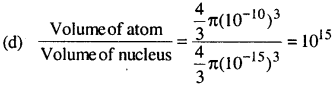Question 8.
(d) The wavelength for Paschen series,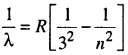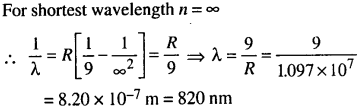Question 9.
(d) Wavelength for Balmer series is $$\frac{1}{\lambda}=R\left(\frac{1}{2^{2}}-\frac{1} at n = ∞, the limit of the series observed.Question 10. (c) The wavelength for Pfund series is given byQuestion 15. (c) Here, λL. = 1215 A, For the first line of Lyman series.Question 20. (a) According to Bohr’s second postulate Angular momentum, [latex]L=\frac{n h}{2 \pi}$$

Angular momentum is also called a moment of momentum.

For second orbit, n = 2 $$L=\frac{2 h}{2 \pi}=\frac{h}{\pi}$$

Question 23.
(c) The radius of nth orbit rn = n2 $$\frac{\hbar^{2} 4 \pi \varepsilon_{0}}{m e^{2}}$$ where $$\frac{\hbar^{2} 4 \pi \varepsilon_{0}}{m e^{2}}=a_{0}[/ltaex] (Bohr radius) Hence, rn = n2 a0. Question 30. (d) Since Bohr’s formula for energy in nth orbit is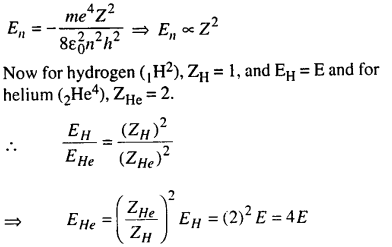Question 31. (d) The energy level diagram schematically looks like this. As we move to higher and higher levels, the energy increases, but the energy gap between any two levels decreases. Hence in the transition from n = 4 to n = 3, energy released will be E4 – E3.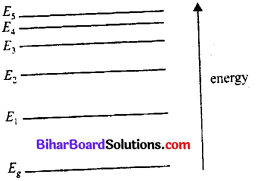And in the transition from n = 2 to n – 1, energy released is E2 -E1. Now as E4 – E3 < E2 – E1 [latex]\Rightarrow h v_{43}<h v_{21} \Rightarrow h v_{43}<h v_{21}$$

Question 32.
(c) As rn = n2 a0
Here, a0 = 0.529 A and n = 3
.-. rn=3 = (3)3 a0 = 9 x 0.529A = 4.76lA

Question 33.
(b) Energy, $$E_{n}=-\frac{13.6}{n^{2}} \mathrm{eV}$$Question 34.
(b) Radius of nlh orbit in hydrogen like atom is
$$r_{n}=\frac{a_{0} n^{2}}{Z}$$

where a0 is the Bohr’s radius For hydrogen atom, Z= 1
∴ r1 = a0 (∵ n = 1 for ground state) 2
For $$\mathrm{Be}^{3+}, \mathrm{Z}=4 ∴ r_{n}=\frac{a_{0} n^{2}}{4}$$
According to given problem $$r_{1}=r_{n} \Rightarrow a_{0}=\frac{n^{2} a_{0}}{4}$$
⇒ n = 2Question 35.
(c) Here the ground state energy, (E) = – 13.6 eV
Since, K.E. of the electron = -E = 13.6 eV
= 13.6 x 1.6 x 10-19 J
= 21.76 x 10-19 J
= 2.18 x 10-18 J.

Question 36.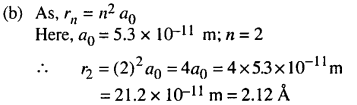Question 37.
(c) Here, = 1, and n2 = 4
Energy of photon absorbed, E = E2 – E1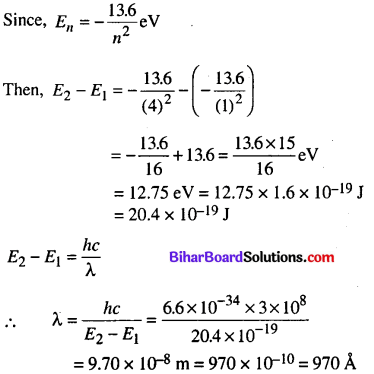Question 38.
(c) According to Bohr’s model $$v=\frac{2 \mathrm{Ke}^{2} \mathrm{Z}}{n h} \text { or } v \propto \frac{1}{n}$$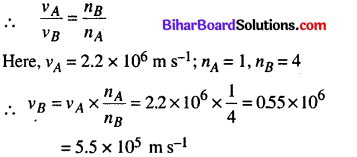Question 39.Question 40.
(c) Speed of the electron in the ground state of hydrogen atom is
$$v=\frac{2 \pi e^{2}}{4 \pi \varepsilon_{0} h}=\frac{c}{137}=c \alpha$$

where, c = speed of light in vacuum, $$\alpha=\frac{e^{2}}{2 \varepsilon_{0} h c}$$ the fine structure constant. It is a pure number whose value is $$\frac{1}{137}$$.

∴ $$\frac{v}{c}=\frac{1}{137}$$Question 41.
(c) The energy needed to remove one electron from the ground state of He = 24.6 eV.
As the He+ is now hydrogen-like, ionisation energy
$$=|-13.6| \frac{2^{2}}{1^{2}} \mathrm{eV} \Rightarrow \mathrm{E}=54.4 \mathrm{eV}$$

∴ To remove both the electrons, energy needed = (54.4 + 24.6) eV = 79. eV

Question 48.
(a) In inelastic collision kinetic energy is not conserved so some part of K.E. is lost.

∴ Reduction in K.E. = K.E. before collision – K.E. after collision
Now, since initial K.E. of each of two hydrogen atoms in ground state = 13.6 eV
Total K.E. of both Hydrogen atom before collision = 2x 13.6 = 27.2 eV
If one H atom goes over to first excited state (n1 = 2) and other remains in ground state (n2 = 1) then their combined K.E. after collision is
$$=\frac{13.6}{(2)^{2}}+\frac{13.6}{(1)^{2}}=3.4+13.6=17 \mathrm{eV}$$
Hence reduction in K.E. = 27.2 – 17 = 10.2 eVAerror: Content is protected !!